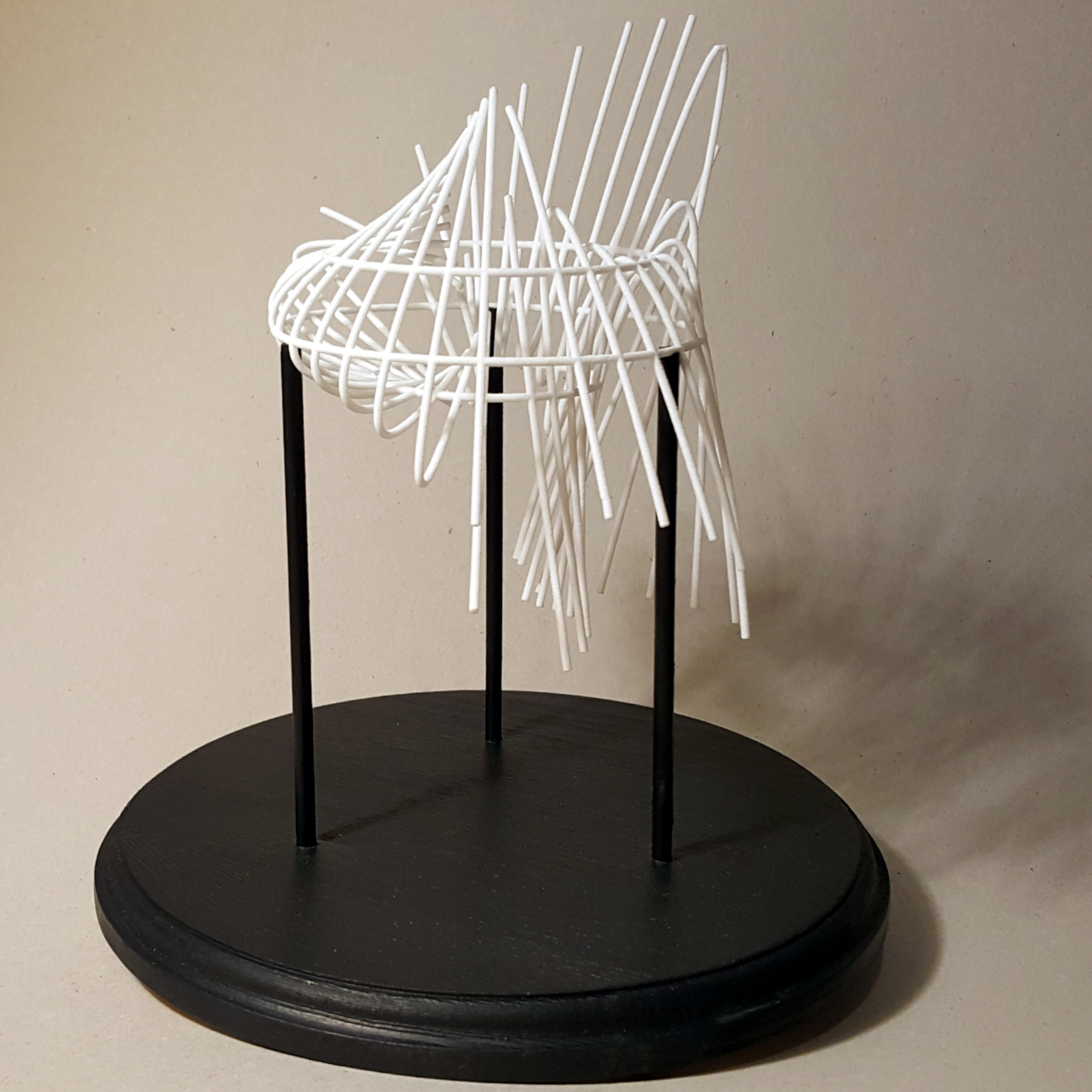Parabolas 3D Printed Plastic, 10 in 2019

This artwork depicts a small sample of the infinite number of parabolas that pass through three points. It is based on a recent paper ("All Parabolas through Three Non-collinear Points" by Huddy and Jones; Mathematical Gazette 102, July 2018, 203-209). Three control points are rotated around the $$z$$-axis resulting in three rings. A parabola exists with an axis of symmetry at every angle $$\theta$$ in the range 0 to $$\pi$$ except the three where the line defined by a pair of the control points is parallel to the axis of symmetry of the corresponding parabola. The parabola with an axis of symmetry at an angle $$\theta$$ is associated with a parabola in the sculpture in the $$xz$$-plane rotated around the $$z$$-axis by an angle twice $$\theta$$.

### References

• "All Parabolas through Three Non-collinear Points" by Huddy and Jones; Mathematical Gazette 102, July 2018, 203-209.